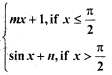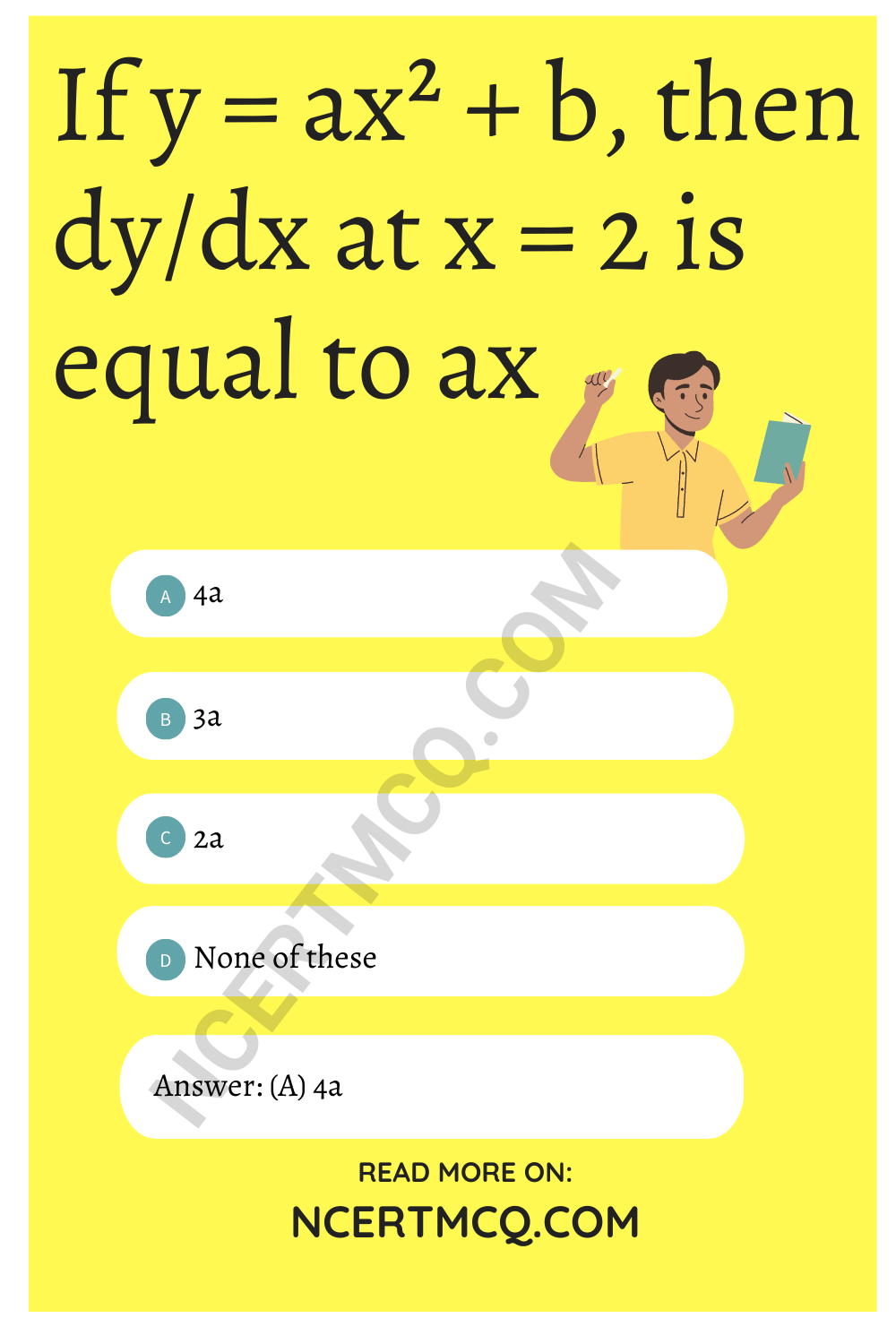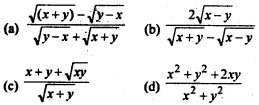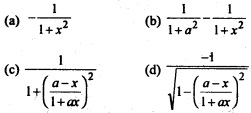Check the below NCERT MCQ Questions for Class 12 Maths Chapter 5 Continuity and Differentiability with Answers Pdf free download. MCQ Questions for Class 12 Maths with Answers were prepared based on the latest exam pattern. We have provided Continuity and Differentiability Class 12 Maths MCQs Questions with Answers to help students understand the concept very well.

## Continuity and Differentiability Class 12 MCQs Questions with Answers

Continuity And Differentiability Class 12 MCQ Question 1.
If f (x) = 2x and g (x) = $$\frac{x^2}{2}$$ + 1, then’which of the following can be a discontinuous function
(a) f(x) + g(x)
(b) f(x) – g(x)
(c) f(x).g(x)
(d) $$\frac{g(x)}{f(x)}$$

Answer: (d) $$\frac{g(x)}{f(x)}$$

Continuity And Differentiability MCQ Question 2.
The function f(x) = $$\frac{4-x^2}{4x-x^3}$$ is
(a) discontinuous at only one point at x = 0
(b) discontinuous at exactly two points
(c) discontinuous at exactly three points
(d) None of these

Answer: (a) discontinuous at only one point at x = 0

Differentiation MCQ Class 12 Question 3.
The set of points where the function f given by f (x) =| 2x – 1| sin x is differentiable is
(a) R
(b) R = {$$\frac{1}{2}$$}
(c) (0, ∞)
(d) None of these

Answer: (b) R = {$$\frac{1}{2}$$}

MCQ On Continuity And Differentiability Question 4.
The function f(x) = cot x is discontinuous on the set
(a) {x = nπ, n ∈ Z}
(b) {x = 2nπ, n ∈ Z}
(c) {x = (2n + 1) $$\frac{π}{2}$$ n ∈ Z}
(d) {x – $$\frac{nπ}{2}$$ n ∈ Z}

Answer: (a) {x = nπ, n ∈ Z}

Class 12 Maths Chapter 5 MCQ Question 5.
The function f(x) = e|x| is
(a) continuous everywhere but not differentiable at x = 0
(b) continuous and differentiable everywhere
(c) not continuous at x = 0
(d) None of these

Answer: (a) continuous everywhere but not differentiable at x = 0

Continuity And Differentiability Class 12 MCQ Term 1 Question 6.
If f(x) = x² sin$$\frac{1}{x}$$, where x ≠ 0, then the value of the function f(x) at x = 0, so that the function is continuous at x = 0 is
(a) 0
(b) -1
(c) 1
(d) None of these

Continuity And Differentiability Class 12 MCQ With Solutions Question 7.
If f(x) =is continuous at x = $$\frac{π}{2}$$, then
(a) m = 1, n = 0
(b) m = $$\frac{nπ}{2}$$ + 1
(c) n = $$\frac{mπ}{2}$$
(d) m = n = $$\frac{π}{2}$$

Answer: (c) n = $$\frac{mπ}{2}$$

MCQ Of Continuity And Differentiability Question 8.
If y = log($$\frac{1-x^2}{1+x^2}$$), then $$\frac{dy}{dx}$$ is equal to
(a) $$\frac{4x^3}{1-x^4}$$
(b) $$\frac{-4x}{1-x^4}$$
(c) $$\frac{1}{4-x^4}$$
(d) $$\frac{-4x^3}{1-x^4}$$

Answer: (b) $$\frac{-4x}{1-x^4}$$

Class 12 Continuity And Differentiability MCQ Question 9.
Let f(x) = |sin x| Then
(a) f is everywhere differentiable
(b) f is everywhere continuous but not differentiable at x = nπ, n ∈ Z
(c) f is everywhere continuous but no differentiable at x = (2n + 1) $$\frac{π}{2}$$ n ∈ Z
(d) None of these

Answer: (b) f is everywhere continuous but not differentiable at x = nπ, n ∈ Z

MCQ Of Differentiation Class 12 Question 10.
If y = $$\sqrt{sin x+y}$$ then $$\frac{dy}{dx}$$ is equal to
(a) $$\frac{cosx}{2y-1}$$
(b) $$\frac{cosx}{1-2y}$$
(c) $$\frac{sinx}{1-xy}$$
(d) $$\frac{sinx}{2y-1}$$

Answer: (a) $$\frac{cosx}{2y-1}$$

MCQ Of Continuity And Differentiability Class 12 Question 11.
The derivative of cos-1 (2x² – 1) w.r.t cos-1 x is
(a) 2
(b) $$\frac{-1}{2\sqrt{1-x^2}}$$
(c) $$\frac{2}{x}$$
(d) 1 – x²

MCQ On Differentiation Class 12 Question 12.
If x = t², y = t³, then $$\frac{d^2y}{dx^2}$$
(a) $$\frac{3}{2}$$
(b) $$\frac{3}{4t}$$
(c) $$\frac{3}{2t}$$
(d) $$\frac{3}{4t}$$

Answer: (b) $$\frac{3}{4t}$$

MCQ On Differentiation Class 12 Pdf Question 13.
The value of c in Rolle’s theorem for the function f(x) = x³ – 3x in the interval [o, √3] is
(a) 1
(b) -1
(c) $$\frac{3}{2}$$
(d) $$\frac{1}{3}$$

MCQ Questions On Differentiation Class 12 Question 14.
For the function f(x) = x + $$\frac{1}{x}$$, x ∈ [1, 3] the value of c for mean value theorem is
(a) 1
(b) √3
(c) 2
(d) None of these

MCQ On Continuity And Differentiability With Solutions Question 15.
Let f be defined on [-5, 5] as
f(x) = {$$_{-x, if x is irrational}^{x, if x is rational}$$ Then f(x) is
(a) continuous at every x except x = 0
(b) discontinuous at everyx except x = 0
(c) continuous everywhere
(d) discontinuous everywhere

Answer: (b) discontinuous at everyx except x = 0

Class 12 Maths Ch 5 MCQ Question 16.
Let function f (x) =(a) continuous at x = 1
(b) differentiable at x = 1
(c) continuous at x = -3
(d) All of these

Class 12 Maths Continuity And Differentiability MCQ Question 17.
If f(x) = $$\frac{\sqrt{4+x}-2}{x}$$ x ≠ 0 be continuous at x = 0, then f(o) =
(a) $$\frac{1}{2}$$
(b) $$\frac{1}{4}$$
(c) 2
(d) $$\frac{3}{2}$$

Answer: (b) $$\frac{1}{4}$$

MCQ Of Chapter 5 Maths Class 12 Question 18.
let f(2) = 4 then f”(2) = 4 then $$_{x→2}^{lim}$$ $$\frac{xf(2)-2f(x)}{x-2}$$ is given by
(a) 2
(b) -2
(c) -4
(d) 3

Ch 5 Maths Class 12 MCQ Question 19.
It is given that f'(a) exists, then $$_{x→2}^{lim}$$ [/latex] $$\frac{xf(a)-af(x)}{(x-a)}$$ is equal to
(a) f(a) – af'(a)
(b) f'(a)
(c) -f’(a)
(d) f (a) + af'(a)

Class 12 Maths Chapter 5 MCQ Questions With Solutions Question 20.
If f(x) = $$\sqrt{25-x^2}$$, then $$_{x→2}^{lim}$$$$\frac{f(x)-f(1)}{x-1}$$ is equal to
(a) $$\frac{1}{24}$$
(b) $$\frac{1}{5}$$
(c) –$$\sqrt{24}$$
(d) $$\frac{1}{\sqrt{24}}$$

Answer: (d) $$\frac{1}{\sqrt{24}}$$

Question 21.
If y = ax² + b, then $$\frac{dy}{dx}$$ at x = 2 is equal to ax
(a) 4a
(b) 3a
(c) 2a
(d) None of theseQuestion 22.
If x sin (a + y) = sin y, then $$\frac{dy}{dx}$$ is equal to
(a) $$\frac{sin^2(a+y)}{sin a}$$
(b) $$\frac{sin a}{sin^2(a+y)}$$
(c) $$\frac{sin(a+y)}{sin a}$$
(d) $$\frac{sin a}{sin(a+y)}$$

Answer: (a) $$\frac{sin^2(a+y)}{sin a}$$

Question 23.
If x $$\sqrt{1+y}+y\sqrt{1+x}$$ = 0, then $$\frac{dy}{dx}$$ =
(a) $$\frac{x+1}{x}$$
(b) $$\frac{1}{1+x}$$
(c) $$\frac{-1}{(1+x)^2}$$
(d) $$\frac{x}{1+x}$$

Answer: (c) $$\frac{-1}{(1+x)^2}$$

Question 24.
If y = x tan y, then $$\frac{dy}{dx}$$ =
(a) $$\frac{tan x}{x-x^2-y^2}$$
(b) $$\frac{y}{x-x^2-y^2}$$
(c) $$\frac{tan y}{y-x}$$
(d) $$\frac{tan x}{x-y^2}$$

Answer: (b) $$\frac{y}{x-x^2-y^2}$$

Question 25.
If y = (1 + x) (1 + x²) (1 + x4) …….. (1 + x2n), then the value of $$\frac{dy}{dx}$$ at x = 0 is
(a) 0
(b) -1
(c) 1
(d) None of these

Question 26.
If f(x) = $$\frac{5x}{(1-x)^{2/3}}$$ + cos² (2x + 1), then f'(0) =
(a) 5 + 2 sin 2
(b) 5 + 2 cos 2
(c) 5 – 2 sin 2
(d) 5 – 2 cos 2

Answer: (c) 5 – 2 sin 2

Question 27.
If sec($$\frac{x^2-2x}{x^2+1}$$) – y then $$\frac{dy}{dx}$$ is equal to
(a) $$\frac{y*2}{x^2}$$
(b) $$\frac{2y\sqrt{y^2-1}(x^2+x-1)}{(x^2+1)^2}$$
(c) $$\frac{(x^2+x-1)}{y\sqrt{y^2-1}}$$
(d) $$\frac{x^2-y^2}{x^2+y^2}$$

Answer: (b) $$\frac{2y\sqrt{y^2-1}(x^2+x-1)}{(x^2+1)^2}$$

Question 28.
If f(x) = $$\sqrt{1+cos^2(x^2)}$$, then the value of f’ ($$\frac{√π}{2}$$) is
(a) $$\frac{√π}{6}$$
(b) –$$\frac{√π}{6}$$
(c) $$\frac{1}{√6}$$
(d) $$\frac{π}{√6}$$

Answer: (b) –$$\frac{√π}{6}$$

Question 29.
Differential coefficient of $$\sqrt{sec√x}$$ is
(a) $$\frac{1}{4√x}$$ = sec √x sin √x
(b) $$\frac{1}{4√x}$$ = (sec√x)3/2 sin√x
(c) $$\frac{1}{2}$$ √x sec√x sin √x.
(d) $$\frac{1}{2}$$√x (sec√x)3/2 sin√x

Answer: (b) $$\frac{1}{4√x}$$ = (sec√x)3/2 sin√x

Question 30.
Let f(x)={$$_{1-cos x, for x ≤ 0}^{sin x, for x > 0}$$ and g (x) = ex. Then the value of (g o f)’ (0) is
(a) 1
(b) -1
(c) 0
(d) None of these

Question 31.
If xmyn = (x + y)m+n, then $$\frac{dy}{dx}$$ is equal to
(a) $$\frac{x+y}{xy}$$
(b) xy
(c) $$\frac{x}{y}$$
(d) $$\frac{y}{x}$$

Answer: (d) $$\frac{y}{x}$$

Question 32.
If $$\sqrt{(x+y)}$$ + $$\sqrt{(y-x)}$$ = a, then $$\frac{dy}{dx}$$Answer: (a) $$\frac{\sqrt{(x+y)}-\sqrt{(y-x)}}{\sqrt{y-x}+\sqrt{x+y}}$$

Question 33.
If ax² + 2hxy + by² = 1, then $$\frac{dy}{dx}$$equals
(a) $$\frac{hx+by}{ax+by}$$
(b) $$\frac{ax+by}{hx+by}$$
(c) $$\frac{ax+hy}{hx+hy}$$
(d) $$\frac{-(ax+hy)}{hx+by}$$

Answer: (d) $$\frac{-(ax+hy)}{hx+by}$$

Question 34.
If sec ($$\frac{x-y}{x+y}$$) = a then $$\frac{dy}{dx}$$ is
(a) –$$\frac{y}{x}$$
(b) $$\frac{x}{y}$$
(c) –$$\frac{x}{y}$$
(d) $$\frac{y}{x}$$

Answer: (d) $$\frac{y}{x}$$

Question 35.
If y = tan-1($$\frac{sinx+cosx}{cox-sinx}$$) then $$\frac{dy}{dx}$$ is equal to
(a) $$\frac{1}{2}$$
(b) $$\frac{π}{4}$$
(c) 0
(d) 1

Question 36.
If y = tan-1($$\frac{√x-x}{1+x^{3/2}}$$), then y'(1) is equal to
(a) 0
(b) ($$\frac{√x-x}{1+x^{3/2}}$$)
(c) -1
(d) –$$\frac{1}{4}$$

Answer: (d) –$$\frac{1}{4}$$

Question 37.
The differential coefficient of tan-1($$\frac{\sqrt{1+x}-\sqrt{1-x}}{\sqrt{1+x}+\sqrt{1-x}}$$) is
(a) $$\sqrt{1-x^2}$$
(b) $$\frac{1}{\sqrt{1-x^2}}$$
(c) $$\frac{1}{2\sqrt{1-x^2}}$$
(d) x

Answer: (c) $$\frac{1}{2\sqrt{1-x^2}}$$

Question 38.
$$\frac{d}{dx}$$[tan-1($$\frac{a-x}{1+ax}$$)] is equal toAnswer: (a) –$$\frac{1}{1+x^2}$$

Question 39.
$$\frac{d}{dx}$$(x$$\sqrt{a^2-x^2}+a^2 sin^{-1}(\frac{x}{a})$$) is equal to
(a) $$\sqrt{a^2-x^2}$$
(b) 2$$\sqrt{a^2-x^2}$$
(c) $$\frac{1}{\sqrt{a^2-x^2}}$$
(d) None of these

Answer: (b) 2$$\sqrt{a^2-x^2}$$

Question 40.
If f(x) = tan-1($$\sqrt{\frac{1+sinx}{1-sinx}}$$), 0 ≤ x ≤ $$\frac{π}{2}$$, then f'($$\frac{π}{6}$$) is
(a) –$$\frac{1}{4}$$
(b) –$$\frac{1}{2}$$
(c) $$\frac{1}{4}$$
(d) $$\frac{1}{2}$$

Answer: (d) $$\frac{1}{2}$$

Question 41.
If y = sin-1($$\frac{√x-1}{√x+1}$$) + sec-1($$\frac{√x+1}{√x-1}$$), x > 0, then $$\frac{dy}{dx}$$ is equal to
(a) 1
(b) 0
(c) $$\frac{π}{2}$$
(d) None of these

Question 42.
If x = exp {tan-1($$\frac{y-x^2}{x^2}$$)}, then $$\frac{dy}{dx}$$ equals
(a) 2x [1 + tan (log x)] + x sec² (log x)
(b) x [1 + tan (log x)] + sec² (log x)
(c) 2x [1 + tan (logx)] + x² sec² (log x)
(d) 2x [1 + tan (log x)] + sec² (log x)

Answer: (a) 2x [1 + tan (log x)] + x sec² (log x)

Question 43.
If y = e3x+n, then the value of $$\frac{dy}{dx}$$|x=0 is
(a) 1
(b) 0
(c) -1
(d) 3e7

Question 44.
Let f (x) = ex, g (x) = sin-1 x and h (x) = f |g(x)|, then $$\frac{h'(x)}{h(x)}$$ is equal to
(a) esin-1x
(b) $$\frac{1}{\sqrt{1-x^2}}$$
(c) sin-1x
(d) $$\frac{1}{(1-x^2)}$$

Answer: (b) $$\frac{1}{\sqrt{1-x^2}}$$

Question 45.
If y = aex+ be-x + c Where a, b, c are parameters, they y’ is equal to
(a) aex – be-x
(b) aex + be-x
(c) -(aex + be-x)
(d) aex – bex

Question 46.
If sin y + e-xcos y = e, then $$\frac{dy}{dx}$$ at (1, π) is equal to
(a) sin y
(b) -x cos y
(c) e
(d) sin y – x cos y

Question 47.
Derivative of the function f (x) = log5 (Iog,x), x > 7 is
(a) $$\frac{1}{x(log5)(log7)(log7-x)}$$
(b) $$\frac{1}{x(log5)(log7)}$$
(c) $$\frac{1}{x(logx)}$$
(d) None of these

Answer: (a) $$\frac{1}{x(log5)(log7)(log7-x)}$$

Question 48.
If y = log10x + log y, then $$\frac{dy}{dx}$$ is equal to
(a) $$\frac{y}{y-1}$$
(b) $$\frac{y}{x}$$
(c) $$\frac{log_{10}e}{x}$$($$\frac{y}{y-1}$$)
(d) None of these

Answer: (c) $$\frac{log_{10}e}{x}$$($$\frac{y}{y-1}$$)

Question 49.
If y = log [ex($$\frac{x-1}{x-2}$$)$$^{1/2}$$], then $$\frac{dy}{dx}$$ is equal to
(a) 7
(b) $$\frac{3}{x-2}$$
(c) $$\frac{3}{(x-1)}$$
(d) None of these

Question 50.
If y = e$$\frac{1}{2}$$ log(1+tan²x), then $$\frac{dy}{dx}$$ is equal to
(a) $$\frac{1}{2}$$ sec² x
(b) sec² x
(c) sec x tan x
(d) e$$\frac{1}{2}$$ log(1+tan²x)

Answer: (c) sec x tan x

Question 51.
If y = 2x32x-1 then $$\frac{dy}{dx}$$ is equal to dx
(a) (log 2) (log 3)
(b) (log lg)
(c) (log 18²) y²
(d) y (log 18)

Question 52.
If xx = yy, then $$\frac{dy}{dx}$$ is equal to
(a) –$$\frac{y}{x}$$
(b) –$$\frac{x}{y}$$
(c) 1 + log ($$\frac{x}{y}$$ )
(d) $$\frac{1+logx}{1+logy}$$

Answer: (d) $$\frac{1+logx}{1+logy}$$

Question 53.
If y = (tan x)sin x, then $$\frac{dy}{dx}$$ is equal to
(a) sec x + cos x
(b) sec x+ log tan x
(c) (tan x)sin x
(d) None of these

Question 54.
If xy = ex-y then $$\frac{dy}{dx}$$ is
(a) $$\frac{1+x}{1+log x}$$
(b) $$\frac{1-log x}{1+log y}$$
(c) not defined
(d) $$\frac{-y}{(1+log x)^2}$$

Answer: (d) $$\frac{-y}{(1+log x)^2}$$

Question 55.
The derivative of y = (1 – x) (2 – x)…. (n – x) at x = 1 is equal to
(a) 0
(b) (-1) (n – 1)!
(c) n ! – 1
(d) (-1)n-1 (n – 1)!

Answer: (b) (-1) (n – 1)!Question 56.
If f(x) = cos x, cos 2 x, cos 4 x, cos 8 x, cos 16 x, then the value of'($$\frac{π}{4}$$) is
(a) 1
(b) √2
(c) $$\frac{1}{√2}$$
(d) 0

Answer: (b) (-1) (n – 1)!

Question 57.
xy. yx = 16, then the value of $$\frac{dy}{dx}$$ at (2, 2) is
(a) -1
(b) 0
(c) -1
(d) None of these

Question 58.
If y = ex+ex+ex+….to∞ find $$\frac{dy}{dx}$$ =
(a) $$\frac{y^2}{1-y}$$
(b) $$\frac{y^2}{y-1}$$
(c) $$\frac{y}{y-1}$$
(d) $$\frac{-y}{y-1}$$

Answer: (c) $$\frac{y}{y-1}$$

Question 59.
If x = $$\frac{1-t^2}{1+t^2}$$ and y = $$\frac{2t}{1+t^2}$$ then $$\frac{dy}{dx}$$ is equal to dx
(a) –$$\frac{y}{x}$$
(b) $$\frac{y}{x}$$
(c) –$$\frac{x}{y}$$
(d) $$\frac{x}{y}$$

Answer: (c) –$$\frac{x}{y}$$

Question 60.
If x = a cos4 θ, y = a sin4 θ. then $$\frac{dy}{dx}$$ at θ = $$\frac{3π}{4}$$ is
(a) -1
(b) 1
(c) -a²
(d) a²

Question 61.
If x = sin-1 (3t – 4t³) and y = cos-1 ($$\sqrt{1-t^2}$$) then $$\frac{dy}{dx}$$ is equal to
(a) $$\frac{1}{2}$$
(b) $$\frac{2}{5}$$
(c) $$\frac{3}{2}$$
(d) $$\frac{1}{3}$$

Answer: (d) $$\frac{1}{3}$$

Question 62.
Let y = t10 + 1 and x = t8 + 1, then $$\frac{d^2y}{dx^2}$$, is equal to
(a) $$\frac{d^2y}{dx^2}$$
(b) 20t8
(c) $$\frac{5}{16t^6}$$
(d) None of these

Answer: (d) $$\frac{1}{3}$$

Question 63.
The derivative of ex3 with respect to log x is
(a) ee3
(b) 3x22ex3
(c) 3x3ex3
(d) 3x2ex3+ 3x2

Question 64.
If x = et sin t, y = etcos t, t is a parameter, then $$\frac{dy}{dx}$$ at (1, 1) is equal to
(a) –$$\frac{1}{2}$$
(b) –$$\frac{1}{4}$$
(c) 0
(d) $$\frac{1}{2}$$

Question 65.
The derivative of sin-1 ($$\frac{2x}{1+x^2}$$) with respect to cos-1 ($$\frac{1-x^2}{1+x^2}$$) is
(a) -1
(b) 1
(c) 2
(d) 4